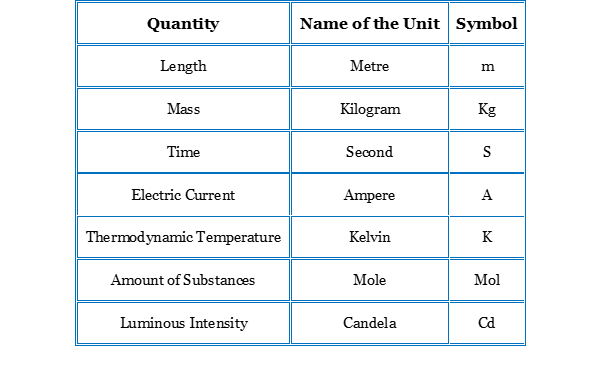# Unit System and Measurement

Hello Everyone! We all know, physics describes the laws of nature. This description is quantitative, and every quantity need to be measured and compared with one another. So, today we are going to learn why Unit System & Measurement is important not only in physics but in all aspects of life?
So, Let’s Start….

A Comparison which makes us understand the quantity

Just imagine, how would we know an elephant is heavier than a goat? And, by how much margin it is heavy? This question can be easily answered if we have chosen a standard mass calling it a unit mass. If the elephant is 250 times the unit mass and the goat is 25 times, we know that the elephant is 10 times heavier than the goat.

If we have the knowledge of unit length and someone says that the Central Park is 5 times the unit length from here, we will have the idea whether should we walk down to the Central Park or should we take an Uber or should we take a train or a flight. Thus, the physical quantities are quantitatively expressed in terms of a unit of that quantity.

It Has two Parts
The measurement of the quantity is mentioned in two parts, the first part gives how many times of the standard unit and the second part gives the name of the unit. Thus, suppose I have to study for 2 hours. The numeric part 2 says that it is 2 times of the unit of time and the second part hour says that the unit chosen here is an hour.
Who Decides the Units?
How is a standard unit chosen for a physical quantity? Think about a weighing scale, how come we know our weight and what is the unit, and who decides the standard unit? Well, here we are going to know the institution that standardizes the units of various physical quantity, accepted by everyone on this planet.
AdvertisementA body named Conference Generale des Poids et Mesures or CGPM also known as General Conference on Weight and Measures in English has been given the authority to decide the units by international agreement. It holds its meetings and any changes in standard units are communicated through the publications of the Conference.
Fundamental and Derived Quantities
There are a large number of physical quantities which are measured, and every quantity needs a definition of unit. However, not all the quantities are independent of each other.
As a simple example, if a unit of length is defined, a unit of area is automatically obtained. If we make a square with its length equal to its breadth equal to the unit length, its area can be called the unit area. All areas can then be compared to this standard unit of area.
Similarly, if a unit of length and a unit of time interval are defined, a unit of speed is automatically obtained. If a particle covers a unit length in unit time interval, we say that it has a unit speed. We can define a set of fundamental quantities as follows:
(a) the fundamental quantities should be independent of each other, and
(b) all other quantities may be expressed in terms of the fundamental quantities.
It turns out that the number of fundamental quantities is only seven. All the rest may be derived from these quantities by multiplication and division. Many different choices can be made for the fundamental quantities. For example, one can take speed and time as fundamental quantities. Length is then a derived quantity.
If something travels at unit speed, the distance it covers in unit time interval will be called a unit distance. One may also take length and time interval as the fundamental quantities and then speed will be a derived quantity.Several systems are in use over the world and in each system the fundamental quantities are selected in a particular way. The units defined for the fundamental quantities are called fundamental units and those obtained for the derived quantities are called the derived units
Fundamental quantities are also called base quantities.

SI Units
In 1971 CGPM held its meeting and decided a system of units which is known as the International System of Units. It is abbreviated as SI from the French name Le Systeme International d’Unites. This system is widely used throughout the world. Following Table gives the fundamental quantities and their units in SI.Fundamental or Base Quantities
Besides the seven fundamental units two supplementary units are defined. They are for plane angle and solid angle. The unit for plane angle is radian with the symbol rad and the unit for the solid angle is steradian with the symbol sr.

SI Prefixes
The magnitudes of physical quantities vary over a wide range. We talk of separation between two protons inside a nucleus which is about 10 -15 m and the distance of a quasar from the earth which is about 1026 m. The mass of an electron is 9.1 x 1031 kg and that of our galaxy is about 2.2 x 1041kg. The CGPM recommended standard prefixes for certain powers of 10. Following Table shows these prefixes.

SI prefixes
 Power of 10 Prefix Symbol Power of 10 Prefix Symbol 18 exa E -1 deci d 15 peta P -2 centi c 12 tera T -3 mili m 9 giga G -6 micro µ 6 Mega M -9 nano n 3 kilo k -12 pico p 2 hecto h -15 femto f 1 deka da -18 atto a
So, this was the Unit System and measurement. I hope you must have liked this post, please follow this blog and comment your valuable feedback and queries.